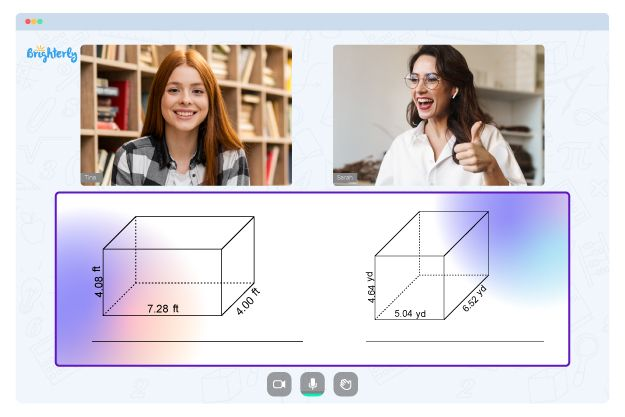# Area Of Parallelogram Worksheets

A parallelogram – as the name implies – is a quadrilateral with two opposite sides being parallel. It’s roughly the same shape as a trapezoid, with only one set of parallel sides. Determining the area of this shape is quite simple for adults but not kids.

Therefore, teachers have developed a modern and effective tool for making parallelogram calculations easier for kids, especially those in the third grade. This tool, called an area of a parallelogram worksheet, contains examples and exercises that kids can easily understand and enjoy.

## The Area of a Parallelogram

A parallelogram is a peculiar shape with four parallel sides and two pairs of opposite sides. In a parallelogram, the opposing sides have the same length, and opposite angles have the same size. And since rectangles and parallelograms have similar properties, the method of finding their areas is the same.

Math for Kids

Is Your Child Struggling With Math?
1:1 Online Math Tutoring## Benefits of an Area of a Parallelogram Worksheet

By finding the area of a parallelogram worksheet and making it available to students, parents have taken the first step toward helping their kids learn. Children will be able to distinguish parallelograms from other geometric shapes. The questions in worksheets will gradually become more difficult – but not overly so – so that children feel comfortable yet motivated when doing math.

Finding the area of a parallelogram worksheets will help students understand how to recognize two parallel lines. They will know how to fill in a missing shape and what a correct parallelogram is. These worksheets are a great interactive way to enhance a kid’s understanding of forms.### Area Of Parallelogram Worksheets PDF

Area Of Parallelogram Worksheet### Area Of Parallelogram Worksheets PDF

Area Of A Parallelogram Worksheet### Area Of Parallelogram Worksheets PDF

Area Of Parallelograms Worksheet### Area Of Parallelogram Worksheets PDF

Parallelogram Area Worksheet

This set of worksheets explains the areas of various parallelograms using side lengths, heights, angles, etc. Here you will find instructions on finding the total size of ​​a parallelogram by dividing it into basic shapes and finding each area. Students will learn how to do this using equations and networks in the area of parallelograms worksheet. Kids gain insight into how these exercises can evolve into real-world engineering calculations.

### Area and Perimeter Worksheets

Struggling with Geometry?• Does your child need extra assistance with mastering geometry?
• Start learning with an online tutor.

Is your child finding it hard to understand geometry lessons? An online tutor could be of help.